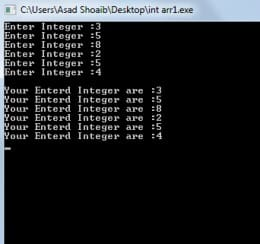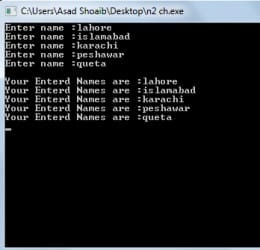Two Dimensional Arrays in C++ with examples

1

In C++ Two Dimensional array in C++ is an array that consists of more than one rows and more than one column. In 2-D array each element is refer by two indexes. Elements stored in these Arrays in the form of matrices. The first index shows a row of the matrix and the second index shows the column of the matrix.

Syntax of Two-Dimensional Array:-

(Data type) (Name of array) [Number of rows] [Number of columns];

For example:-

Int matrix  ;

When we type the above statement the compiler will generate a 2-D array of a matrix which consists of 7 rows and 7 columns.

Accessing each location of Two Dimensional Arrays:-

In order to store values in a C++ two dimensional arrays the programmer have to specified the number of row and the number of column of a matrix. To access each individual location of a matrix to store the values the user have to provide exact number of row and number of column.

For Example:-

int matrix ;

matrix   = 43;
matrix   = 44;
matrix   = 56;
matrix   = 72;

How to enter data in a Two Dimensional Arrays:-

Nested loop is used to enter data in 2-D arrays. It depends upon the programmer which loop he wants to use it could be While loop or it could be a For loop. The outer loop acts as the number of rows of a matrix and the inner loop acts as the number of columns of a matrix.

For Example:-

#include<iostream.h>
#include<conio.h>

main()
{

int matrix  ;
for (int m1=0 ; m1<5 ; m1++)
{
for (int m2=0 ; m2<5 ; m2++)
{
matrix [m1] [m2] = 5 ;
}
}
getch();
}

The above portion of a code uses nested For loop to assigns 5 to all the locations of a matrix of a two dimensional array in C++.

How to initialize Two-Dimensional Integer Array:-

The 2-D arrays which are declared by the keyword int and able to store integer values are called two dimensional integer arrays. The process of assigning values during declaration is called initialization. TheseArrays can be initialized by putting the curly braces around each row separating by a comma also each element of a matrix should be separated by a comma.

Example of a Two Dimensional Integer Array:-

int mat = {
{ 3,6,8 },
{ 5,4,7 },
{ 2,4,7 }
};As you can see the above array is declared by a keyword int therefore it is a 2-D integer array.

Now consider a following example of a complete program which will elaborate the working.

#include<iostream.h>
#include<conio.h>

main()
{
int matrix ;
// For taking integer inputs in a matrix //
for (int m1=0 ; m1<2 ; m1++)
{
for (int m2=0 ; m2<3 ; m2++)
{
cout<<"Enter Integer :";
cin>>matrix [m1][m2];
}
}
cout<<endl;

// For displaying elements of a matrix on a screen //
for (int m1=0 ; m1<2 ; m1++)
{
for (int m2=0 ; m2<3 ; m2++)
{
cout<<"Your Entered Integer are :";
cout<<matrix [m1][m2];
cout<<endl;
}
}
getch();
}

Explanation:-

In a main function first of all the two dimensional integer array will be declared by the name of a matrix, then the integer elements will be stored in an array with the help of a nested For loop. Then again with the help of another nested loop the program will print the elements stored in the matrix on a computer screen.

/* */

How to initialize Two-Dimensional Character Array:-

The 2-D arrays which are declared by the keyword char and able to store characters are called two dimensional character arrays. 2-D characterArray can be initialized by putting the curly braces around each row as well as apostrophe around every alphabet.

Example of Two-Dimensional Character Array:-

As you can see the above array is declared by a keyword int therefore it is a 2-D character array.

Now consider a following example of a complete program which will elaborate the working of character array.

char bravo  = { 'c', 'a', 't' ,
'b', 'a', 't' ,
'g', 'a', 't' };As you can see the above array is declared by a keyword int therefore it is a two dimensional character array.

Now consider a following example of a complete program which will elaborate the working of character array.

#include<iostream.h>
#include<conio.h>

main()
{
// the number of columns should be greater than the length of a name otherwise compiler will produce errors //
char cmatrix ;
// For taking integer inputs in a matrix //
for (int q1=0 ; q1<5 ; q1++)
{
for (int m2=0 ; m2<15 ; m2++)
{
cout<<"Enter name :";
cin>>cmatrix [q1];
}
}
cout<<endl;

// For displaying elements of a matrix on a screen //
for (int q1=0 ; q1<5 ; q1++)
{
for (int m2=0 ; m2<15 ; m2++)
{
cout<<"Your Enterd Names are :";
cout<<cmatrix [q1];
cout<<endl;
}
}

getch();
}

Explanation:-

[yop_poll id=”3″]

In a main function 2-D character arrays will be declared by the name of a cmatrix. Then the character elements will be stored in an array with the help of a nested for loop. Then another nested loop will come into action to print the elements stored in the cmatrix on a computer screen.

In case of a two dimensional character array to take an input and produce an output on the screen we only have to specify row of a cmatrix.

If you like my article on two dimensional arrays in C++ then please provide your feedback, your feedback is appreciated.

If you like my work and wanted to read my other articles then visit my Homepage.

@ 2014 HellGeeks.com

Two Dimensional Arrays in C++ with examples
4.6 (92%) 5 vote[s](royal52) is a software engineer. He is good at authoritative link building, Content Writing, SEO, Web Development, Wordpress, HTML, CSS, JAVASCRIPT, Game Development, Unity3d, Graphic Designing, Logo Designing, Android Development and Ios Development. Last but not least, he is passionate about development and gaming.

1. Reply• Reply